Email Us: info@lupinepublishers.comCall Us: +1 (914) 407-6109   57 West 57th Street, 3rd floor, New York - NY 10019, USA

# Lupine Publishers Group

## Lupine Publishers

Submit Manuscript

ISSN: 2643-6744# Comparison between the Second Zagreb Eccentricity Index and Eccentric Connectivity Index of Graphs Volume 1 - Issue 1

Huimin Li1, Fang Gao1 and Kexiang Xu ay2*

• 1College of Science, China
• 2School of Mathematics and Computer Science, China

Received: November 12, 2018;   Published: November 19, 2018

*Corresponding author: Kexiang Xu ay, School of Mathematics and Computer Science, Anhui 247000, PR China

DOI: 10.32474/CTCSA.2018.01.000105

## Abstract

For a graph G, the second Zagreb eccentricity index E2(G) and eccentric connectivity index ∈c(G) are two eccentricity-based invariants of graph G. In this paper we prove some results on the comparison between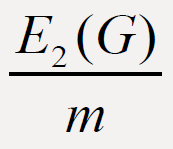andof connected graphs G of order n and with m edges.

The authors demonstrated how a combination of both techniques and human interventions enhances control, decision-making and data analysis systems.

Keywords: Graph; Eccentricity (of vertex); Second Zagreb eccentricity index; Eccentric connectivity index

## Introduction

Throughout this paper we only consider the note, undirected, simple and connected graphs. The degree of v∈ V(G), denoted by degG(v), is the number of vertices in G adjacent to v. For any two vertices u; v in a graph G, the distance between them, denoted by dG(u; v), is the length of a shortest path connecting them in G. As usual, let Sn, Pn, Cn, Kn be the star graph, path graph, cycle graph and complete graph, respectively, on n vertices. Other undefined notations and terminology on the graph theory can be found in . For any vertex of graph G, the eccentricity ∈G (v) (or ∈(v) for short) is the maximum distance from v to other vertices of G, i.e., ∈G (v)= maxu≠v dG(u,v). The eccentricity of a vertex is an important parameter in pure graph theory. The radius of a graph G is denoted by r(G) and defined by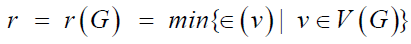. Also, the diameter of G, denoted by d(G), is the maximum distance between vertices of a graph G and hence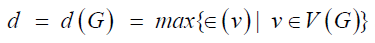. A vertex v with ∈G(v)= r(G) is called a central vertex in G. A graph G with d(G) = r(G) is called a self-centered graph. A graph which contains only two non-central vertices is called almost self-centered graph  (ASC graph for short). Moreover, the eccentricity is also applied in chemical graph theory. There are several eccentricity-based topological indices, including the second Zagreb eccentricity index E2(G)  and eccentric connectivity index ∈c (G) , of graphs G where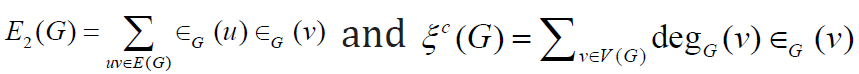In particular, we haveor any graph G. In this paper we prove some comparison results betweenandof connected graphs G of order n with m edges. Main results In this we prove several results on the comparison betweenandof graphs G. Firstly we present two useful lemmas.

Lemma 2.1:  Let G be a connected graph of order n with maximum degree Δ . If Δ= n −1 then E2(G) =ξc(G) .Otherwise, E2(G) ≥ξc(G)with equality holds if and only if G is a 2-SC graph.

Lemma 2.1:  If u and v are two adjacent vertices of a connected graph G, then ∈(𝒰)−∈(𝒱) |≤1.

Denote by Gn(m; d) the set of connected graphs of order n with m edges and diameter d.

Theorem 2.3. Let G∈ζ(𝓂,𝒹) with n>5 and 𝒹≤2. Then<. Proof. If d = 1, G∈ζ(𝓂,𝒹) contains a single graph Kn with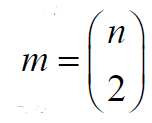and. Then our result follows. Next it suffices to consider the case when d = 2. If G has maximum degree Δ = n −1by Lemma 2.1, we have E2(G) <ξc(G) for any graph G∈ζ(𝓂,𝒹) .Moreover, we have 𝓂≥𝓃−1 If 𝓂=n-1, then G≅Sn with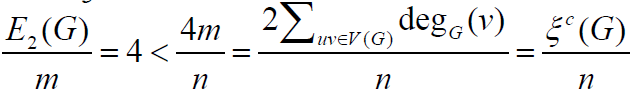for any n ≥ 5. Moreover,<holds clearly form ≥ n. If Δ ≤ n − 2 then G is a 2-SC graph. By Lemma 2.2, G is never a tree. Therefore m ≥ n with equality holding if and only if G ≅ C4 or G ≅ C5 . Consider that n > 5, m > n holds immediately. It follows that. This completes the proof of the theorem.

In the following we consider the graphs G∈ζ(𝓂,𝒹) with diameter d ≥ 3.

Theorem 2.4: Let G∈ζ(𝓂,𝒹). with d ≥ 3, n > 5 be a tree or a unicyclic graph. Then>Proof. If d ≥ 3, then Δ(G) ≤ n − 2 . From Lemma 2:1, we have E2(G) <ξc(G). Note that m ≥ n for any tree or unicyclic graph G. Thus, it follows. finishing the proof of the theorem. Next we consider the case

m > n. In the following theorem we give a sufficient condition for the graph G of order n with.

Theorem 2.5. Let G∈ζ(𝓂,𝒹) with d ≥ 3, m = n + t and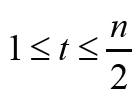. If r(G) ≥ 3, then,

Proof. Making a difference, we have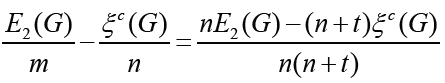Set Δ1= nE2(G)−(n + t)ξc(G) . From Lemma 2.2, we have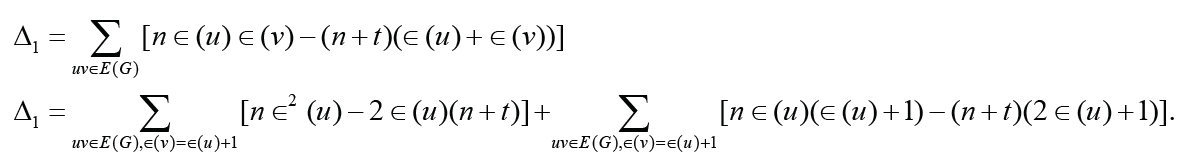Since r(G) ≥3 and, we have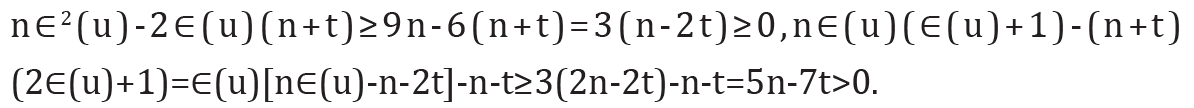Therefore, Δ1 ≥ 0 with equality holding if and only if ∈(𝓊) = 3for each vertex 𝓊∈V(G) that is, G is a self-centered graph with radius 3. This completes the proof of the theorem.

F or, G∈ζ(𝓂,𝒹) with d ≥ 3, r = 2 andconsidering that r(G)≤d(G)≤2r(G) we have d(G) = 3 or d(G) = 4. In this case, the value of Δ1 may be negative, zero or positive. Let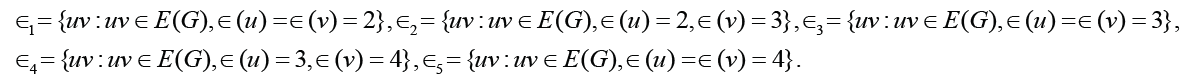Denote by mi the cardinality of i∈{1, 2,3, 4,5}. ThenIn the following result we present some comparison results for ASC graphs.

Theorem 2.6: Let G∈ζ(𝓂,𝒹) with d = 3, r = 2, m = n + t, t ≥ 1 where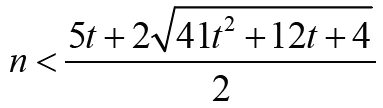.

If G is an ASC graph, then<Proof. If G is an ASC graph with d = 3, r = 2, from the structure of ASC graph, we have 𝓂3≤ 𝓃 − 2,𝓂3=0, that is, 1 𝓂 ≥ t + 2 . If 𝓃 ≤ 5t, clearly, we have Δ1 ≤ 0 .For n >

5t, we have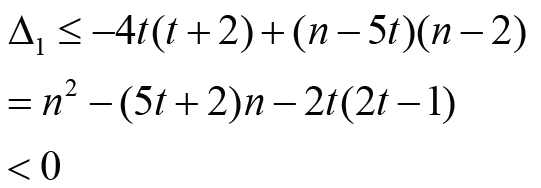holds if and only if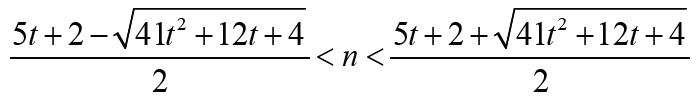Note that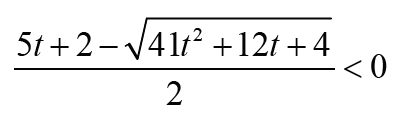Thus Δ1 < 0 is equivalent thatwith t ≥1. Therefore the result holds immediately. It is much interesting to search more generalized graphs G with different comparison results betweenandwhich can be a topic for further research in the future.

Close## Online Submission System

### Submit ManuscriptClick here for Manuscript Sample Templates### Drag and drop files here

or

( For multiple files submission, zip them in a single file to submit. For file zipping software Download )